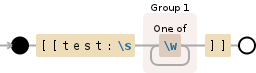# Get a particular list of String using Regex in java [closed]

My string will look like this,

``````lopsakf pkpsdkf pskadp fkpsdkfp sdaf

[[test: lls]]
[[test: mmdm]]
[[test: owow]]
[[test: www]]
[[test: wowow]]
``````

I would like to get the values lls, askd, mmdm, etc.. and store it in a List. Please note that there are huge volume of such texts. I need an efficient way to parse each set and store it in a List,without using any external libraries.

• "I need an.." ..demonstration of (any) effort, as well as a question. – Andrew Thompson Sep 5 '13 at 0:37

You can try the regular expression:

``````(?<=\[\[test: )[^]]+
``````

In ahother hand, you need to use a constant of `java.util.regex.Pattern` for avoid recompiled the expression every time, something like that:

``````private static final Pattern REGEX_PATTERN =
Pattern.compile("(?<=\\[\\[test: )[^]]+");

public static void main(String[] args) {

Matcher matcher = REGEX_PATTERN.matcher(input);
while (matcher.find()) {
System.out.println(matcher.group());
}
}
``````

Output:

``````lls
mmdm
owow
www
wowow
``````

Use `matcher.find()` and `matcher.group()` for get the required strings.

In another way, use a instance of `java.util.ArrayList` with an initial capacity already defined, according to the number of strings that may exist, so that the list should not be required copy the elements to a new internal array.

``````\[\[test:\s([\w]+)\]\]
``````Edit live on Debuggex

it essentially captures `[[test: *any number and character here* ]]` in a capture group which generally returns as an array.

NOTE: you maybe need to escape characters (I.E. \\[)

Something like the below using a `Matcher` to extract each string and add it to a list should work (regex is untested):

``````String input = "My input string....";

List<String> myStrings = new ArrayList<String>();
String pattern = "\\[\\[test: (\\w+)\\]\\]";
Matcher matcher = Pattern.compile(pattern).matcher(input);
while (matcher.find())
{
String matchedString = matcher.group(1);
You could also add to a `Set` instead of a `List` if you want to avoid duplicate strings.